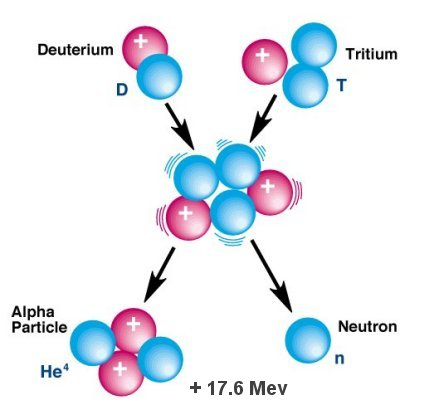What is Nuclear Fusion?

Nuclear Fusion is described as a process where in two nuclei are combined in order to form a heavy nucleus. An example of two nuclei of heavy hydrogen is 1H2 fuse to form nucleus of helium, which is 2He3. During the process of nuclear fusion, the product mass formed is somewhat lesser compare to reactant mass. This is identified as Mass Defect, on the other hand the missing mass is change to energy based on Einstein’s equation of mass energy, which is E = mc2.  Once there are two deuterium nuclei combine there are twp reactions that could be formed.

The two reactions formed in combining two deuterium nuclei combine

• 1H2 + 1H2 → 2He3 + 0H1 + 3.3 MeV
• 1H2 + 1H2 → 1H3 + 1H1 + 4.0 MeV

The reaction above acquires major advantage and that is the setting of about 33 grams of deuterium from 1000 liter of seawater. Hence, seawater has about 1015 tons of deuterium. A gallon of deuterium discharge energy, which is equivalent to the one discharged by 600 gallons of petrol. The reaction 1H3 + 1H2 → 2He4 + 0n1 + 17.6 MeV, which involves tritium is probable at low temperature and offer more energy. The tritium proportion in seawater is less, however it could be obtained through bombarding lithium isotope with one neutron, which is shown in the reaction 3Li6 + 0n1 → 1H3 + 2He4
and 3Li7 + 0n1 → 1H3 + 2He4 + 0n1.In the process of thermonuclear fusion, a fusion fuel is heat up to 106 K when ionization occurs to create plasma. Plasma is the combination of moving charged particles having an equal number of positive and then negative charges. It is totally hard to control thermonuclear fusion, but there must be attempts that need to be done in order to obtain controlled fusion process to get energy. The uncontrolled nuclear fusion is employed to create mass destruction weapons for instance the hydrogen bomb. Comparing to nuclear fission, nuclear fusion acquires advantages.